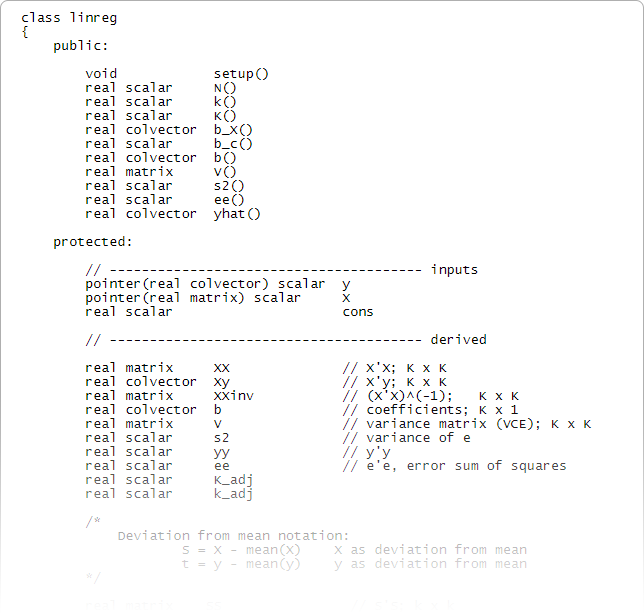Home  /  Mata

## Mata—Stata's serious programming language

Mata is a programming language that looks a lot like Java and C but adds direct support for matrix programming. Mata is a compiled language, which makes it fast. You can use Mata interactively when you want to quickly perform matrix calculations, or you can use Mata when you need to write complex programs. Mata has the structures, pointers, and classes that you expect in your programming language. In fact, Mata is Stata's development language. Most new features of Stata are written in Mata. This includes multilevel modeling, latent class analysis, Bayesian estimation, and even the core algorithms of the graphical SEM Builder. But Mata is not just for Stata developers; you too can take advantage of this powerful programming language.

You can use Mata interactively.

A = 1,2\3,4		    // Input a 2 × 2 matrix
b = -1\3		    // Input a 2 × 1 column vector
A'b			    // Compute A'b
A[2,1]			    // Access the (2,1) element of A
A[,2]      		    // Access the second column of A
B = diag(b)		    // Create a 2 × 2 matrix with the elements of b on the diagonal
C = A,B     		    // Combine matrices A and B by column
D = A\B     		    // Or by row
I = I(3)		    // Create a 3 × 3 identity matrix I
trace(A)		    // Compute the trace of A
det(A)			    // And the determinant of A
G*H			    // Multiply G (n × k) by H (k × k)
G:*g  			    // Multiply each column of G (n × k)  by  g (n × 1)
st_data(.,.)                // Load Stata dataset into Mata
st_data((1,5\7,9), "mpg")   // Observations 1 to 5 and 7 to 9 of variable mpg


Or you can write a simple program for regression.

mata
st_view(y=., ., "mpg")
st_view(X=., ., ("weight", "foreign", "cons"))
b = invsym(X'X)*X'y
b
e = y - X*b
n = rows(X)
k = cols(X)
s2= (e'e)/(n-k)
V = s2*invsym(X'X)
V
se = sqrt(diagonal(V))
(b, se)


Or build a regression system using structures or classes.### Resources

There are many great resources for learning Mata.

The official documentation:

We recommend starting with the intro sections:

The Mata Matters articles published in the Stata Journal:

Programming an estimation command in Stata, a series of posts on the Stata Blog.

An Introduction to Mata from the Social Science Computing Cooperative at the University of Madison–Wisconsin

Estimation of the Mixture of Gaussians Using Mata, blog post by Dallakyan Aramayis.